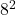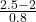## The time it takes 5th graders to complete a particular mathematics problem are Normally distributed with a mean 2 minutes and a standard dev

Question

The time it takes 5th graders to complete a particular mathematics problem are Normally distributed with a mean 2 minutes and a standard deviation of 0.8 minutes.
a. Find the probability that a randomly chosen 5th grader will take more than 2.5 minutes to complete the problem.

in progress 0
2 months 2021-07-19T16:56:40+00:00 1 Answers 1 views 0

0.266

Step-by-step explanation:

x  ~ N(2,0.)

P(x>2.5)

=P(Z>)

=p(z >0.625)

=1-
Φ(0.625)

= 1 – 0.734

=0.266

Hope this helps please make me brainliest!